# Class 10 Maths Coordinate Geometry NCERT Solutions Exercise 7.3

In this page we have Class 10 Maths Coordinate Geometry NCERT Solutions for Exercise 7.3 . Hope you like them and do not forget to like , social_share and comment at the end of the page.
Question 1 Find the area of the triangle whose vertices are
(i) (2, 3), (–1, 0), (2, – 4) (ii) (–5, –1), (3, –5), (5, 2)
Question 2. In each of the following find the value of ‘k’, for which the points are collinear.
(i) (7, –2), (5, 1), (3, k) (ii) (8, 1), (k, – 4), (2, –5)
Question 3. Find the area of the triangle formed by joining the mid-points of the sides of the triangle whose vertices are (0, –1), (2, 1) and (0, 3). Find the ratio of this area to the area of the given triangle.
Question 4. Find the area of the quadrilateral whose vertices, taken in order, are (– 4, – 2), (– 3, – 5), (3, – 2) and (2, 3).
Question 5. You have studied in Class IX, (Chapter 9, Example 3), that a median of a triangle divides it into two triangles of equal areas. Verify this result for Δ ABC whose vertices are A (4, – 6), B (3, –2) and C (5, 2).

Solution 1
Area of triangle ABC of coordinates A(x1,y1), B(x2,y2) and C(x3,y3)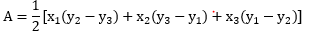i) Area of the given triangle = 1/2 [2 {0- (-4)} + (-1) {(-4) - (3)} + 2 (3 - 0)]
= 1/2 {8 + 7 + 6}
= 21/2 square units.
(ii) Area of the given triangle = 1/2 [-5 {(-5)- (4)} + 3(2-(-1)) + 5{-1 - (-5)}]
= 1/2{35 + 9 + 20}
= 32 square units
Solution2
Area of triangle ABC of coordinates A(x1,y1) , B(x2,y2) and C(x3,y3)For point A, B and C to be collinear, the value of A should be zero
(i) For collinear points, area of triangle formed by them is zero.
1/2 [7 {1- k} + 5(k-(-2)) + 3{(-2) + 1}] = 0
7 - 7k + 5k +10 -9 = 0
-2k + 8 = 0
k = 4
(ii) For collinear points, area of triangle formed by them is zero.
1/2 [8 { -4- (-5)} + k {(-5) -(1)} + 2{1 -(-4)}] = 0
8 - 6k + 10 = 0
6k = 18
k = 3

Solution 3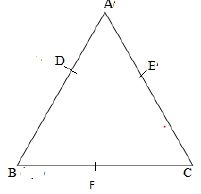Let the vertices of the triangle be A (0, -1), B (2, 1), C (0, 3).
Let D, E, F be the mid-points of the sides of this triangle.
Coordinates of D, E, and F are given by mid-point formula
D = [(0+2)/2, (-1+1)/2] = (1,0)
E = [(0+0)/2, (-3-1)/2] = (0,1)
F = [(2+0)/2, (1+3)/2] = (1,2)
Now we know that
Area of triangle of coordinates A(x1,y1) , B(x2,y2) and C(x3,y3) is given by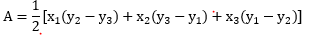So Area of ΔDEF = 1/2 {1(2-1) + 1(1-0) + 0(0-2)}
= 1/2 (1+1) = 1 square units
Area of ΔABC = 1/2 [0(1-3) + 2{3-(-1)} + 0(-1-1)]
= 1/2 {8} = 4 square units
Therefore, the required ratio is 1:4.

Solution 4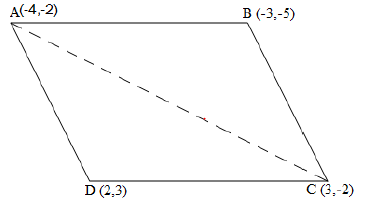Let the vertices of the quadrilateral be A (- 4, - 2), B (- 3, -5), C (3, - 2), and D (2, 3). Join AC to form two triangles ABC and ΔACD.
Now we know that
Area of triangle of coordinates (x1,y1) , (x2,y2) and (x3,y3) is given by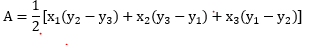Area of ΔABC = 1/2 [(-4) {(-5) - (-2)} + (-3) {(-2) - (-2)} + 3{(-2) - (-5)}]
= 1/2 (12+0+9)
= 21/2 square units
Area of ΔACD = 1/2 [(-4) {(-2) - (3)} + 3{(3) - (-2)} + 2 {(-2) -(-2)}]
= 1/2 (20+15+0)
= 35/2 square units
Area of ΔABCD = Area of ΔABC + Area of ΔACD
= (21/2 + 35/2) square units = 28 square units
Solution 5
Let the vertices of the triangle be A (4, -6), B (3, -2), and C (5, 2).
Let D be the mid-point of side BC of ΔABC. Therefore, AD is he median in ΔABC.
Coordinates of point D (midpoint of B &C) = (3+5/2, -2+2/2) = (4,0)
Now we know that
Area of triangle of coordinates (x1,y1) , (x2,y2) and (x3,y3) is given byArea of ΔABD = 1/2 [(4) {(-2) - (0)} + 3{(0) - (-6)} + (4) {(-6) - (-2)}]
= 1/2 (-8+18-16)
= -3 square units
However, area cannot be negative. Therefore, area of ΔABD s 3 square units.
Area of ΔADC 1/2 [(4) {0 - (2)} + 4{(2) - (-6)} + (5) {(-6) -(0)}]
= 1/2 (-8+32-30)
= -3 square units
However, area cannot be negative. Therefore, area of ΔADC is 3 square units.
The area of both sides is same. Thus, median AD has divided ΔABC in two triangles of equal areas

Reference Books for class 10

Given below are the links of some of the reference books for class 10 math.

You can use above books for extra knowledge and practicing different questions.

### Practice Question

Question 1 What is $1 - \sqrt {3}$ ?
A) Non terminating repeating
B) Non terminating non repeating
C) Terminating
D) None of the above
Question 2 The volume of the largest right circular cone that can be cut out from a cube of edge 4.2 cm is?
A) 19.4 cm3
B) 12 cm3
C) 78.6 cm3
D) 58.2 cm3
Question 3 The sum of the first three terms of an AP is 33. If the product of the first and the third term exceeds the second term by 29, the AP is ?
A) 2 ,21,11
B) 1,10,19
C) -1 ,8,17
D) 2 ,11,20

Note to our visitors :-

Thanks for visiting our website.
DISCLOSURE: THIS PAGE MAY CONTAIN AFFILIATE LINKS, MEANING I GET A COMMISSION IF YOU DECIDE TO MAKE A PURCHASE THROUGH MY LINKS, AT NO COST TO YOU. PLEASE READ MY DISCLOSURE FOR MORE INFO.